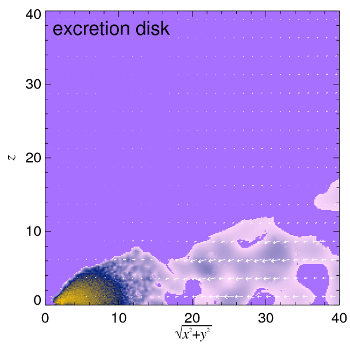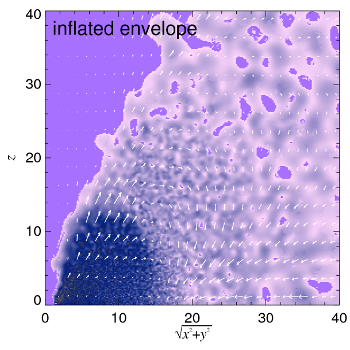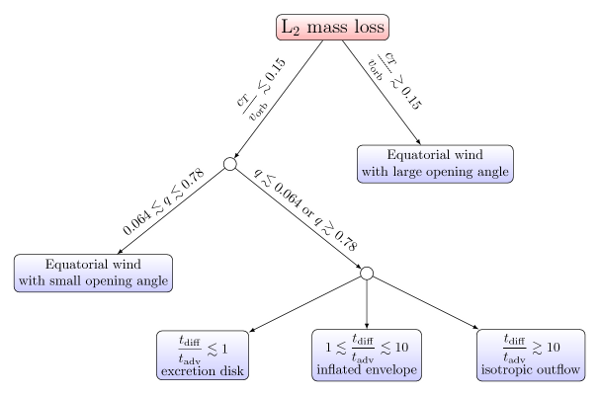## Binary Stellar Mergers with Marginally-Bound Ejecta: Excretion Disks, Inflated Envelopes, Outflows, and their Luminous Transients

### O. Pejcha, B. D. Metzger, and K. Tomida

#### Link to our paperWe study the radiative hydrodynamics of mass loss from the outer Lagrange point (L2) of binary stars in the context of merger events and their luminous transients. Previously, we showed that synchronous L2 mass loss for binary mass ratios 0.06 < q < 0.8 results in a radiatively inefficient dust-forming unbound equatorial outflow. Here, we find that similar outflows exist irrespective of q if the initial ratio of gas sound speed cT to the orbital speed vorb is ε = cT/vorb > 0.15. For cold L2 mass-loss (ε < 0.15) from binaries with q < 0.06 or q > 0.8, the marginally-bound equatorial outflow falls back on a timescale of tens to hundreds of binary orbits, and experiences additional tidal torqueing and shocking. The virialization of the gas with the binary orbit leads to a slow luminosity increase at approximately constant size of the photosphere. Further evolution depends on the efficiency of radiative cooling. If the ratio of radiative diffusion to advection time is tdiff/tadv < 1, the virialized gas collapses to an excretion disk, while for tdiff/tadv > 10 an isotropic wind is formed. In between these two extremes, an inflated envelope transports the heat generated near the binary to the surface by meridional flows. In all cases, the radiated luminosity reaches ~10-2 to 10-1 of Ṁ vorb2/2. We discuss the implications for transients in the luminosity gap between classical novae and supernovae such as V1309 Sco and V838 Mon.#### Here we present links to all videos relevant for our paper.

Each video shows density, temperature, velocity, luminosity, effective temperature, average specific angular momentum, and the unbound mass. Unless explicitly noted, the simulations assume constant T=4500 K and ε = 0.05 at L2, =1000/P, inflow inner boundary condition, and the full complement of physics discussed in the paper. Some of the videos have only frames at the same absolute orientation of the binary to reduce the length.

= 10 M/yr = 1 M/yr = 10-1 M/yr = 10-2 M/yr = 10-3 M/yr = 10-4 M/yr
a = 0.03 AU, M1 = 0.8 M, M2 = 0.9 M link link link
no diff, no cool
no diff, no cool, reflecting
a = 0.2 AU, M1 = 4.0 M, M2 = 4.5 M link link link
a = 0.03 AU, M1 = 0.1 M, M2 = 2.0 M link link link
a = 0.2 AU, M1 = 0.8 M, M2 = 0.9 M link link link
a = 1.3 AU, M1 = 0.8 M, M2 = 0.9 M link link
a = 0.0045 AU, M1 = 0.8 M, M2 = 0.9 M link link